• 操作系统文件管理实验代码

千次阅读 2020-12-22 18:08:36
实现了简单的文件系统操作。 没有实现磁盘块之间的连接，目录与磁盘属于一对一链接，没法指定磁盘块存放并且文件内容超出磁盘块就无法存储了。 #include<iostream> #include<string> using namespace ...

实现了简单的文件系统的操作。
没有实现磁盘块之间的连接，目录与磁盘属于一对一链接，没法指定磁盘块存放并且文件内容超出磁盘块就无法存储了。

#include<iostream>
#include<string>
using namespace std;

const int MaxsizeOfIndex = 10;			//目录的大小
const int MaxsizeOfDiskBlock = 2000;	//磁盘块的大小

//磁盘
typedef struct Disk {
char disk[MaxsizeOfDiskBlock];		//磁盘块中存储的内容
};

//目录
typedef struct Index{
int empty;				//当前目录是否有文件
char name;			//文件名
};

Disk d[MaxsizeOfIndex];									//磁盘块号
Index f[MaxsizeOfIndex];								//目录

//函数的声明
void create();
void Delete();
void open();
void write();
void delete_content();
void show_file_system();

int main() {
int op_type;   //操作种类

//文件系统初始化，全部标记为未使用
for (int i = 0; i < MaxsizeOfIndex; i++) {
f[i].empty = 0;
}

//文件系统操作
while (1) {
cout << "1.创建文件" << " 2.删除文件" << " 3.读文件" << " 4.写入文件" << " 5.添加文件内容" <<" 6.删除文件内容" <<" 7.结束" << endl;
cout << "请输入：";
cin >> op_type;			//输入操作码
switch (op_type)
{
case 1:create(); break;
case 2:Delete(); break;
case 3:open(); break;
case 4:write(); break;
case 6:delete_content(); break;
default:
break;
}
if (op_type == 7)
break;
show_file_system();
}
return 0;
}

//创建文件(创建索引)
void create() {
int state = 0;										//标记是否正常创建文件,后面所有state均为标记，标记功能是否正常运行
char write_state = 'N';                             //写入标记，创建完文件询问是否需要直接写入
char Delete_state = 'N';                            //删除标记，空间不足，询问是否删除
for (int i = 0; i < MaxsizeOfIndex; i++) {
if (f[i].empty == 0) {							//找到空区域
f[i].empty = 1;								//标记为已用
cout << "请输入文件名：";					//输入想要创建的文件的名称
cin >> f[i].name;
state = 1;                                  //标记为正常创建
cout << "是否要输入文件内容（Y/N):";		//询问创建之后要不要输入
cin >> write_state;
if (write_state == 'Y') {
cout << "请输入文件内容：";             //为了不用重复输入文件名，这里没有调用write()，感觉可以使用同名函数
cin >> d[i].disk;
}
break;
}
}
if (state == 0) {									//没有空区域，询问是否删除
cout << "没有剩余空间，删除文件（Y/N）：";
cin >> Delete_state;
if (Delete_state == 'Y') {
Delete();
}

}

}

//删除文件(删除索引)
void Delete() {											//将此处标记为空，删除索引，不用删除内容
char name;
int state = 0;
char Delete_state;
cout << "请输入想删除的文件名：";					//输入需要删除的文件名
cin >> name;
for (int i = 0; i < MaxsizeOfIndex; i++) {
if (strcmp(f[i].name, name) == 0 && f[i].empty == 1) {	//找到同名文件，且块为占用块
f[i].empty = 0;								//标记为空
cout << "删除成功" << endl;
state = 1;									//标记正常删除文件索引
break;
}
}
if (state == 0) {                                   //未正常删除
cout << "删除失败，请输入正确的文件名,重新输入(Y/N): ";
cin >> Delete_state;
if (Delete_state == 'Y') {
Delete();
}
}
}

//打开文件
void open() {
char name;
char write_state;
char empty_cmp = { '\0' };
cout << "请输入文件名要打开的：";								//输入想要打开的文件名
cin >> name;
for (int i = 0; i < MaxsizeOfIndex; i++) {
if (strcmp(f[i].name, name) == 0 && f[i].empty == 1) {		//名字相同，且块不为空，因为删除的时候，不会删除其中的名字，只是标记为空
if (strcmp(d[i].disk, empty_cmp) == 0) {				//块内无内容，询问要不要先写入
cout << "文件内容为空，请先写入文件，写入(Y/N):"<<endl;
cin >> write_state;
if (write_state == 'Y') {
write();
}
}
else {													//块内有内容，直接读出
cout << "文件内容为：" << endl;
cout << d[i].disk << endl;
}
break;

}
}
}

//写入文件
void write() {
char name;
int state = 0;
char write_state = 'N';
cout << "请输入文件名：";
cin >> name;
for (int i = 0; i < MaxsizeOfIndex; i++) {
if (strcmp(f[i].name, name) == 0 && f[i].empty == 1) {		//找到已经建立索引的文件
cout << "请输入文件内容：";
cin >> d[i].disk;										//将内容写入到对应的磁盘块中
state = 1;												//标记为正常写入
break;
}
}
if (state == 0) {												//写入失败
cout << "写入失败，请输入正确的文件名,重新输入(Y/N): ";
cin >> write_state;
if (write_state == 'Y') {
write();
}
}
}

//添加文件内容
char content;
char name;
int char_site;
int size;
cout << "请输入想操作的文件：";
cin >> name;
cout << "想要添入的内容：";
cin >> content;
cout << "请输入想添加到的位置：";
cin >> char_site;
for (int i = 0; i < MaxsizeOfIndex; i++) {
if (strcmp(f[i].name, name) == 0 && f[i].empty == 1) {
int temp = i;
for (int i = sizeof(d[temp].disk)-1; i > char_site ; i--) {    //向后挪出空间
d[temp].disk[i + strlen(content)] = d[temp].disk[i];
}
for (int i = 0; i < strlen(content); i++) {   //这里不能使用sizeof，因为sizeof包括了最后的'\0'，会导致输出认为到这里就结束了
d[temp].disk[i + char_site] = content[i];
}
}
}
cout << "写入成功";
}

//删除文件内容
void delete_content() {
char name;
int state = 0;
char Delete_state = 'N';
cout << "请输入想要删除的文件内容的文件名：";
cin >> name;
for (int i = 0; i < MaxsizeOfIndex; i++) {
if (strcmp(f[i].name, name) == 0 && f[i].empty == 1) {
memset(d[i].disk, '\0', sizeof(d[i].disk));			//把磁盘块设置为空
cout << "删除完成";									//正常删除
state = 1;
break;
}
}
if (state == 0) {											//删除失败
cout << "删除失败，请输入正确的文件名,重新输入(Y/N): "<<endl;
cin >> Delete_state;
if (Delete_state == 'Y') {
delete_content();
}
}
}

void show_file_system() {
int state = 0;
cout << "文件号" << "	" << "文件名" <<endl;
for (int i = 0; i < MaxsizeOfIndex; i++) {
if (f[i].empty == 1) {
state = 1;
cout << i + 1 << "	" << f[i].name << endl;
}
}
if (state == 0) {
cout << "无文件" << endl;
}
}

展开全文c++ 操作系统
• 目录操作系统实验四 文件管理实验内容运行环境实验原理源代码验证数据c库函数实现复制文件系统调用函数实现复制文件模拟ls-l 功能运行截图c语言库函数实现复制文件系统调用函数实现复制文件模拟ls -l功能 ...

实验内容

1. 利用库函数fopen(), fread(), fwrite(), fclose() 实现简单的文件备份， 即将一个文件的内容拷贝到另一个文件中去。
2. 利用系统调用函数open(), read(), write(), close() 实现上述功能。

VM 15 pro
ubuntu 20.04

实验原理

使用C库函数来实现简单文件备份的原理：

在linux系统中，文件和设备都被看做事数据流，进行操作之前，必须先将流打开，可以通过调用库函数fopen()打开一个流，库函数fopen()的返回值为一个FILE结构指针，此结构中包含对所打开的流进行操作所需的全部信息。当流操作完成后，需要执行清空缓冲区，保存数据等操作，所以这个时候需要将流关闭，调用函数fclose()来完成。在实现文件备份的过程中，实际上是建立了两个流，把其中一个流中的数据写入另一个流中从而实现备份。

使用系统调用函数实现简单文件备份的原理：

先从文件1中读取数据，并建立文件2，然后将文件1中的数据写入文件2，关闭文件1，关闭文件2。

两者对比：

系统调用open()等函数和C库函数fopen()等函数都是对文件的操作的相关函数，但前者是在内核模式中运行的，且是在文件描述符的基础上进行，而后者则是在用户模式下运行的，且是在数据流的基础上进行，而每一个文件流都对应一个底层的文件描述符。在进行C库函数fopen()等函数的调用时，相应的操作会在进入内核中以相应系统调用函数实现。

源代码

验证数据

//filecopy-data.dat
1906300151 yj

c库函数实现复制文件

// filecopy.c
#include<sys/types.h>
#include<stdio.h>
#include<stdlib.h>

int main()
{
char buf;
FILE *source, *backup;
if ((source = fopen("./filecopy-data.dat", "r"))==NULL)
{
printf("Error in opening file.\n");
exit(1);
}
if ((backup = fopen("./filecopy-cdata.dat", "w"))==NULL)
{
printf("Error in creating file.\n");
exit(1);
}
while (fread(&buf, sizeof(buf), 1, source) == 1)
{
if (!fwrite(&buf, sizeof(buf), 1, backup))
{
printf("Error in writing file.\n");
exit(1);
}
}
if (ferror(source) != 0)
{
exit(1);
}
else
{
}
if (fclose(source))
{
printf("Error in close file.\n");
exit(1);
}
else
{
}

if (fclose(backup))
{
printf("Error in close file.\n");
exit(1);
}
else
{
printf("success in close file.\n");
exit(1);
}
}

系统调用函数实现复制文件

//filecopy1.c
#include <sys/types.h>
#include <sys/stat.h>
#include <unistd.h>
#include <fcntl.h>
#include <stdio.h>
#include <stdlib.h>
#include <string.h>

int main(int argc,char* argv[]){
int fd,fd1,fd2;
char bufs;
int len;
if(argc != 3){
printf("error in argc!\n");
}else{
fd = open(argv,O_RDONLY|O_CREAT);
if(fd != -1){
fd1 = creat(argv,0775);
if(fd1 != -1){
fd2 = open(argv,O_WRONLY);
write(fd2,bufs,len);
printf("successfully copied!\n");
}
}else{
printf("erorr in opening!\n");
}
}else{
printf("erorr in opening!\n");
}
}
return 0;
}

模拟ls-l 功能

//ls.c
#include <stdio.h>
#include <stdlib.h>
#include <unistd.h>
#include <sys/stat.h>
#include <sys/types.h>
#include <string.h>
#include <time.h>
#include <pwd.h>
#include <grp.h>
#include <time.h>
#include <dirent.h>

#define MAX_FILE_NUM 200

typedef struct LS
{
char mode;	// 文件的模式
int dir_num;	// 是否目录或目录中包含目录的数量
char user;	// 文件的用户名
char group;	// 文件的组名
long size;		// 文件的字节数
char time;	// 文件的最后修改时间
char year;	// 拓展用，年份
char mon;	// 月份
char hour;	// 时
char min;	// 分
int st_mode;	// 文件类型和权限
char name;	// 文件名
}LS;

// 获取文件的模式
char* file_mode(mode_t m,char* str)
{
if(S_ISREG(m))
str = '-';
else if(S_ISDIR(m))
str = 'd';
else if(S_ISCHR(m))
str = 'c';
else if(S_ISBLK(m))
str = 'b';
else if(S_ISFIFO(m))
str = 'q';
else if(S_ISLNK(m))
str = 'l';
//	else if(S_ISSOCK(m))
//		str = 's';
else
str = '?';

str = '\0';

strcat(str,S_IRUSR&m?"r":"-");
strcat(str,S_IWUSR&m?"w":"-");
strcat(str,S_IXUSR&m?"x":"-");

strcat(str,S_IRGRP&m?"r":"-");
strcat(str,S_IWGRP&m?"w":"-");
strcat(str,S_IXGRP&m?"x":"-");

strcat(str,S_IROTH&m?"r":"-");
strcat(str,S_IWOTH&m?"w":"-");
strcat(str,S_IXOTH&m?"x":"-");

return str;
}

// 获取目录的数量
int dir_count(char* path)
{
DIR *dir;
dir = opendir(path);
struct dirent *dirent;
int count = 0;
{
if(dirent->d_type == 4)
count++;
}
closedir(dir);
return count;
}

// 是否是目录或目录下有目录
int is_dir(struct dirent *dirent)
{
char* a = dirent->d_name;
if(dirent->d_type == 8)
return 1;
if(dirent->d_type == 4)
{
if(dir_count(a) == 0)
return 2;
else
return dir_count(a);
}
}

// 获取用户名
char* file_user(uid_t st_uid,char* str)
{
struct passwd *user;
user = getpwuid(st_uid);
sprintf(str,"%s",user->pw_name);
return str;
}

// 获取组名
char* file_group(uid_t st_uid,char* str)
{
struct passwd *user;
user = getpwuid(st_uid);
struct group *grp;
grp = getgrgid(user->pw_gid);
sprintf(str,"%s",grp->gr_name);
return str;
}

// 获取文件大小
off_t file_size(struct stat buf)
{
off_t size = buf.st_size;
return size;
}

// 获取最后修改时间
char* file_time(time_t mt,char* str)
{
struct tm* t = localtime(&mt);
sprintf(str,"%d月 %02d %02d:%02d",t->tm_mon+1,t->tm_mday,t->tm_hour,t->tm_min);
return str;
}

// 获取文件的数量
int file_count(char* path)
{
DIR *dir;
dir = opendir(path);
struct dirent *dirent;
int count = 0;
{
count++;
}
closedir(dir);
return count;
}

// 交换
void equal(LS *a,LS *b)
{
strcpy(a->mode,b->mode);
a->dir_num = b->dir_num;
strcpy(a->user,b->user);
strcpy(a->group,b->group);
a->size = b->size;
strcpy(a->time,b->time);
a->st_mode = b->st_mode;
strcpy(a->name,b->name);
}

// 排序
void sort(LS *info,int index)
{
LS *temp = (LS*)malloc(sizeof(LS));
for(int i=index-1; i>0; i--)
{
for(int j=0; j<i; j++)
{
if(strcmp(info[i].name,info[j].name)<0)
{
equal(temp,&info[i]);
equal(&info[i],&info[j]);
equal(&info[j],temp);
}
}
}
}

// 输出结构体
void show_ls(LS *info,int index)
{
for(int i=0; i<index; i++)
{
//printf("%d: ",i);
printf("%s \033[0m",info[i].mode);
printf("%d ",info[i].dir_num);
printf("%s ",info[i].user);
printf("%s ",info[i].group);
printf("%5ld ",info[i].size);
printf(" %s ",info[i].time);
//printf("%d ",info[i].st_mode);
if(16893 == info[i].st_mode)
{
// 颜色
printf("\033[34m\033[1m%s\033[0m",info[i].name);
}
else if(33277 == info[i].st_mode)
{
printf("\033[32m\033[1m%s\033[0m",info[i].name);
}
else
{
printf("%s",info[i].name);
}
if(i < index)
printf("\n");
}
//printf("循环结束\n");
}

// 创建结构体，赋值
LS *create(struct stat buf,struct dirent *dirent)
{
LS* info = (LS*)malloc(sizeof(LS));
char str = {};
//puts(file_mode(buf.st_mode,str));
strcpy(info->mode,file_mode(buf.st_mode,str));
//puts(info->mode);
info->dir_num = is_dir(dirent);
strcpy(info->user,file_user(buf.st_uid,str));
strcpy(info->group,file_group(buf.st_uid,str));
info->size = file_size(buf);
strcpy(info->time,file_time(buf.st_mtime,str));
info->st_mode = buf.st_mode;
strcpy(info->name,dirent->d_name);

return info;
}

int main(int argc,char* argv[])
{
LS info[MAX_FILE_NUM];
char* l = "-l";
if(argc != 2)
{
printf("仅支持传入 -l\n");
return 1;
}
if(strcmp(argv,l) != 0)
{
printf("\"ls：无法识别的选项\"%s\"\n",argv);
printf("请尝试执行\"ls --help\"来获取更多信息。\n");
return 0;
}
char* a = ".";
char* b = "..";
char* path = malloc(10000);
strcpy(path,"./");	// 只支持当前路径
int count = file_count(path);

DIR *dir;
dir = opendir(path);
struct dirent *dirent;
int index = 0;	// 结构体下标
int blocks = 0;
for(int i=0; i<count; i++)
{
struct stat buf = {};
if(stat(dirent->d_name,&buf))
{
perror("stat");
return -1;
}

// 跳过特殊情况
if(strcmp(dirent->d_name,a)==0 || strcmp(dirent->d_name,b)==0)
continue;
blocks += buf.st_blocks;
//printf("%d\n",blocks);
info[index++] = *create(buf,dirent);
}
closedir(dir);
//printf("文件总数:%d\n",index);
//show_ls(info,index);

printf("总用量 %d\n",blocks/2);
sort(info,index);
show_ls(info,index);
return 0;
}

运行截图

复制前filecopy-data.dat文件与内容：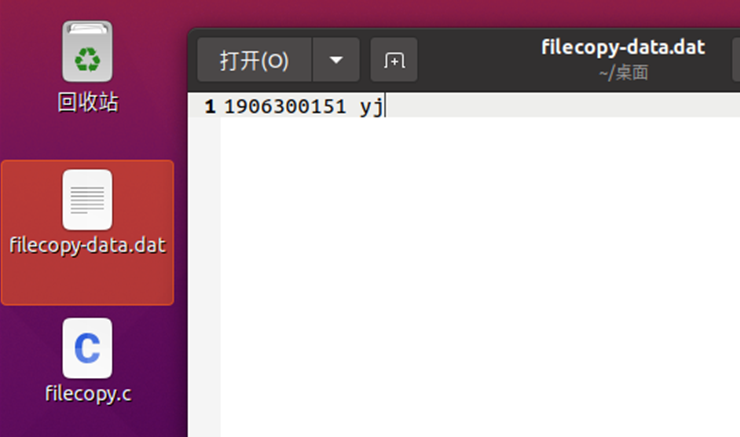c语言库函数实现复制文件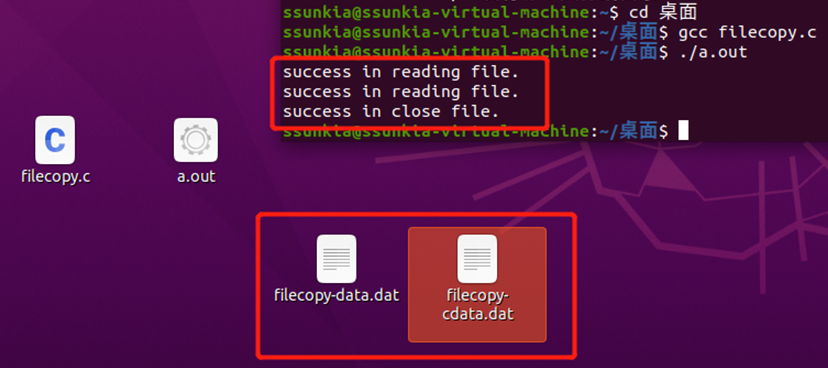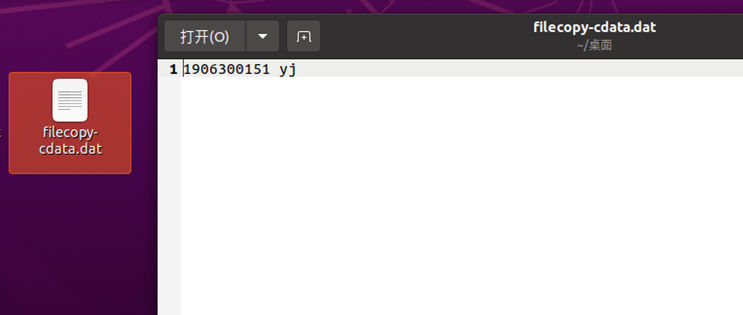系统调用函数实现复制文件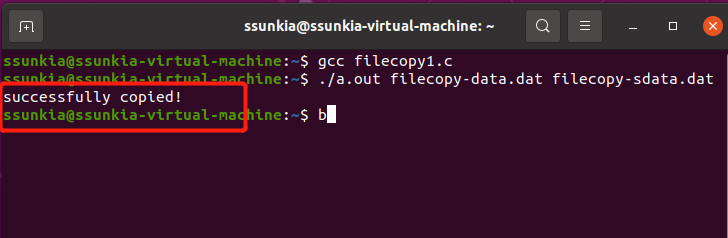模拟ls -l功能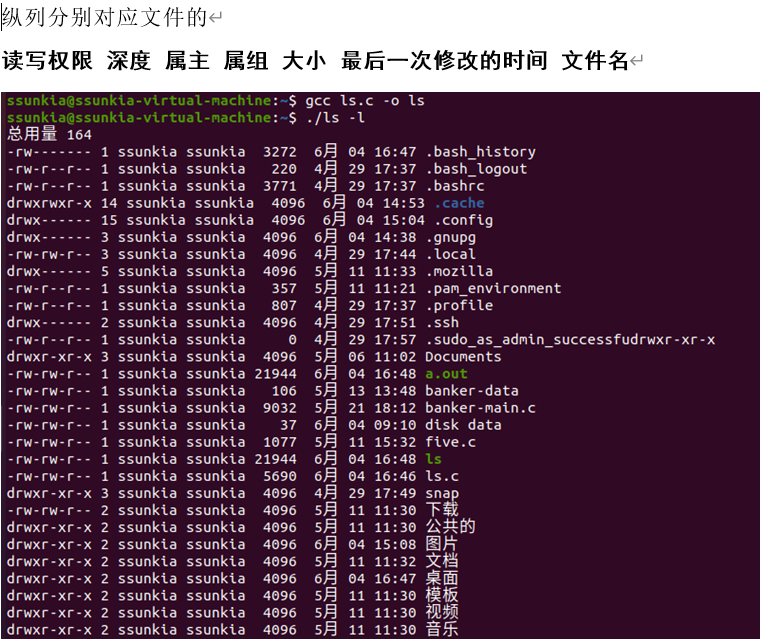展开全文操作系统
• 操作系统实验五：文件管理

千次阅读 2021-01-21 17:51:50

操作系统期末复习总结（SUES版）：

实验五 文件管理

一、实验要求
（1）了解文件权限及作用；
（2）掌握文件权限的设置；
（3）掌握文件权限的管理与应用；
（4）掌握文件基本操作；
（5）理解文件系统管理文件的实现机制。
二、实验内容
（1）验证实验：
① 在用户主目录下创建目录test，进入test目录创建空文件file1。并以长格形式显示文件信息，注意文件的权限和所属用户和组；
② 在目录test中, 新建文件file2，设置文件权限为r–r-----。再新建文件test2，查看其属性，要求设置权限为-rw-------；
③ 查看目录test及其中文件的所属用户和组。 把目录test及其下的所有文件的所有者改成bin，所属组改成daemon。查看设置结果。 删除目录test及其下的文件;
④ 查看/etc/inittab文件的权限属性，并指出该文件的所有者以及文件所属组群;
⑤ 查找/etc目录下以i开头的文件和目录；
⑥ 查找root用户所有以t开头的文件，并将其保存在/root/tmp文件中；
⑦ 在/root下建立/etc/fstab的符号链接文件；
⑧ 新建文件test，分别为其建立硬链接文件和符号链接文件。指出硬链接文件的索引号与符号链接文件的索引号的差异；
⑨ 在/usr目录下创建一个目录usr_test和文本文件test，并建立一个test文件的链接test02。通过修改test文件中的内容查看test和test02中内容的情况，并分析原因。
（2）编程实验
实现一个简单的文件系统，具体要求如下：
① 内存中开辟一块虚拟磁盘空间作为文件存储分区，在其上实现一个简单的基于多级目录的单用户单任务系统中的文件系统；
② 在退出该文件系统的使用时，虚拟文件系统以一个文件的方式保存到磁盘中，以便下次可以把它恢复到内存的虚拟存储空间；
③ 能根据绝对路径和相对路径进行文件查找。
三、实验结果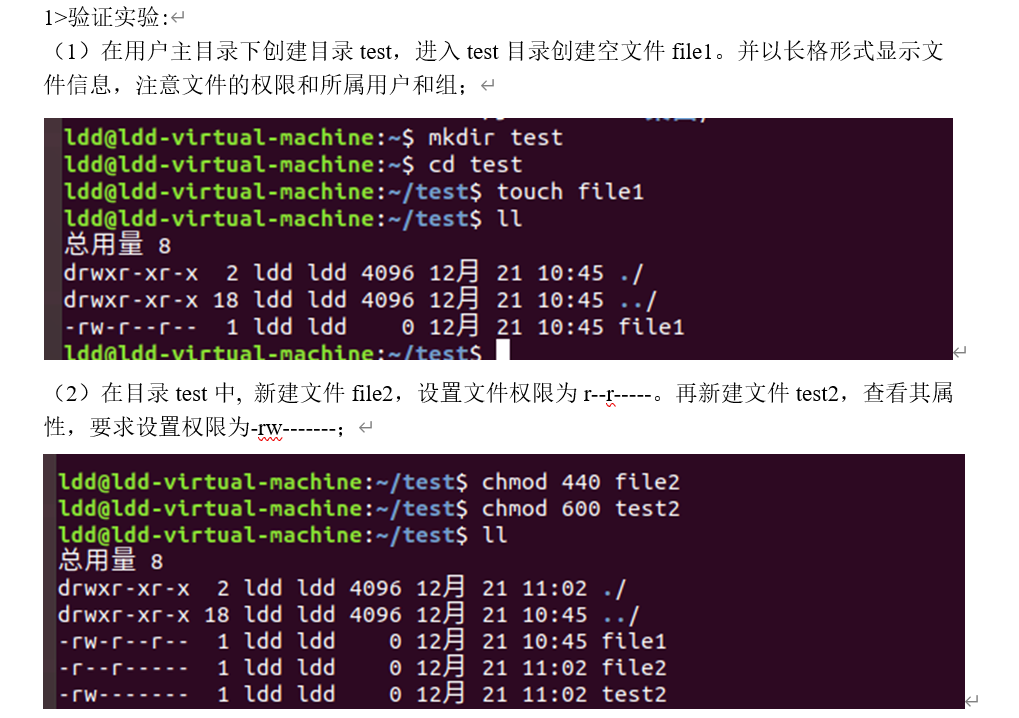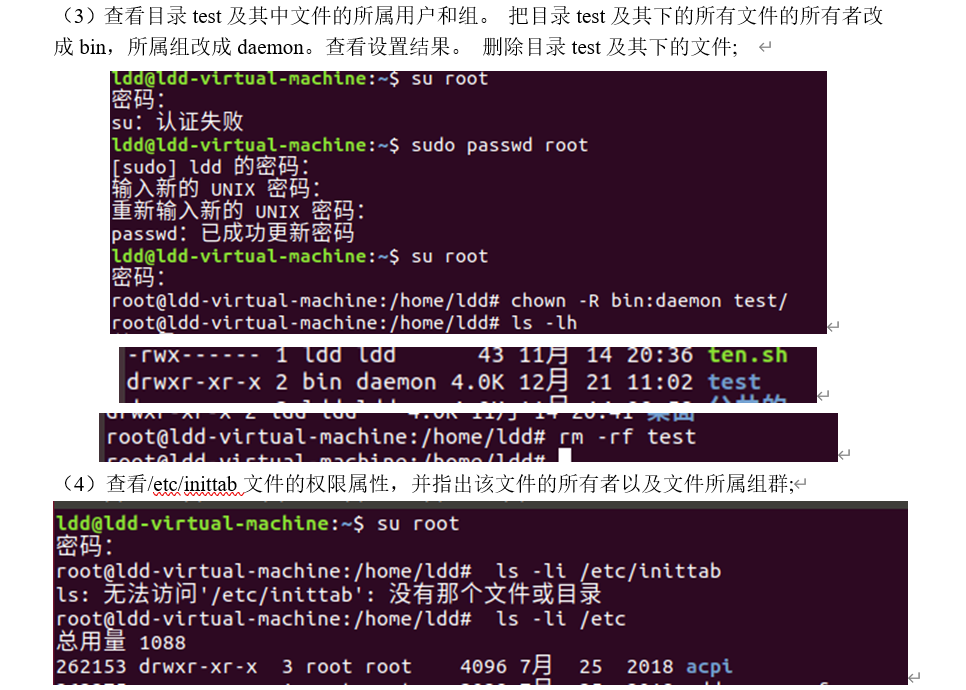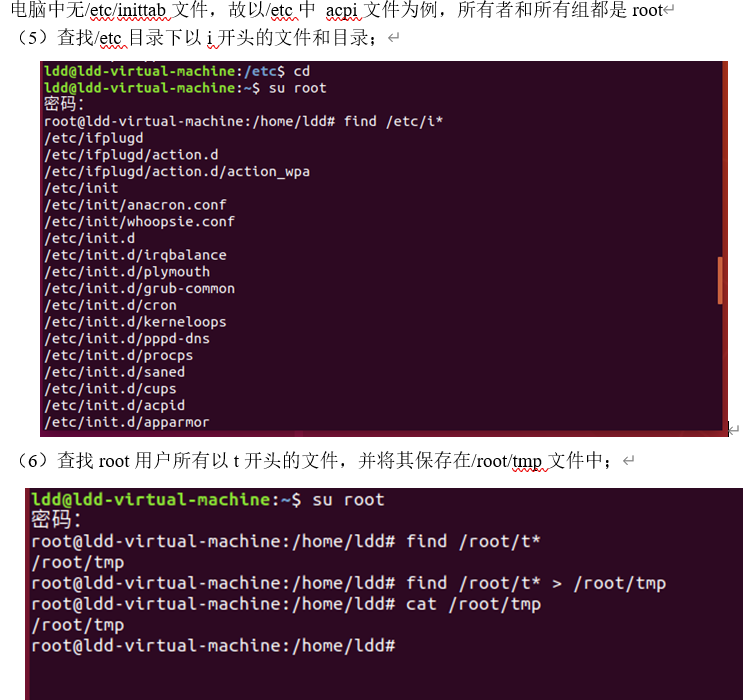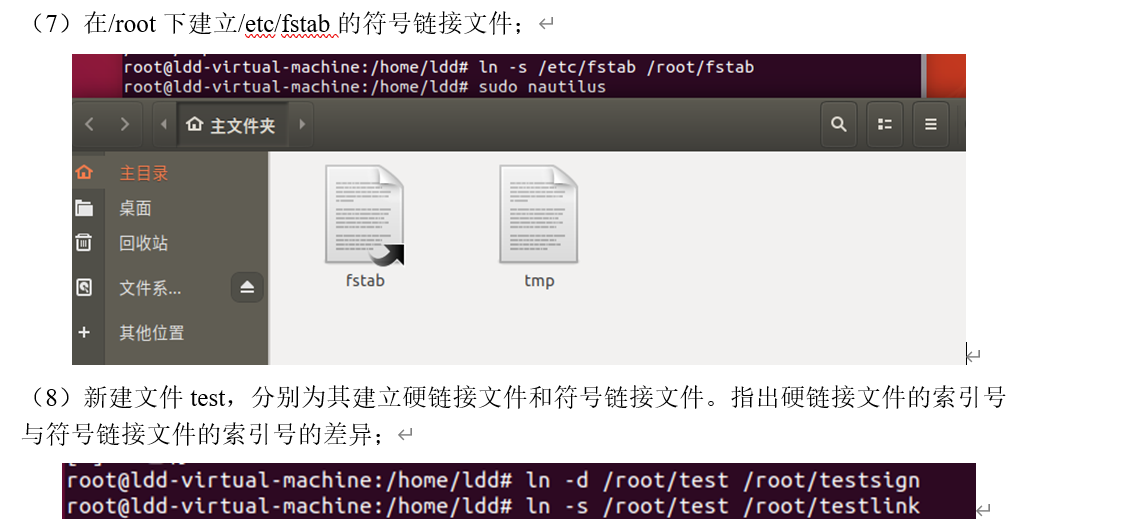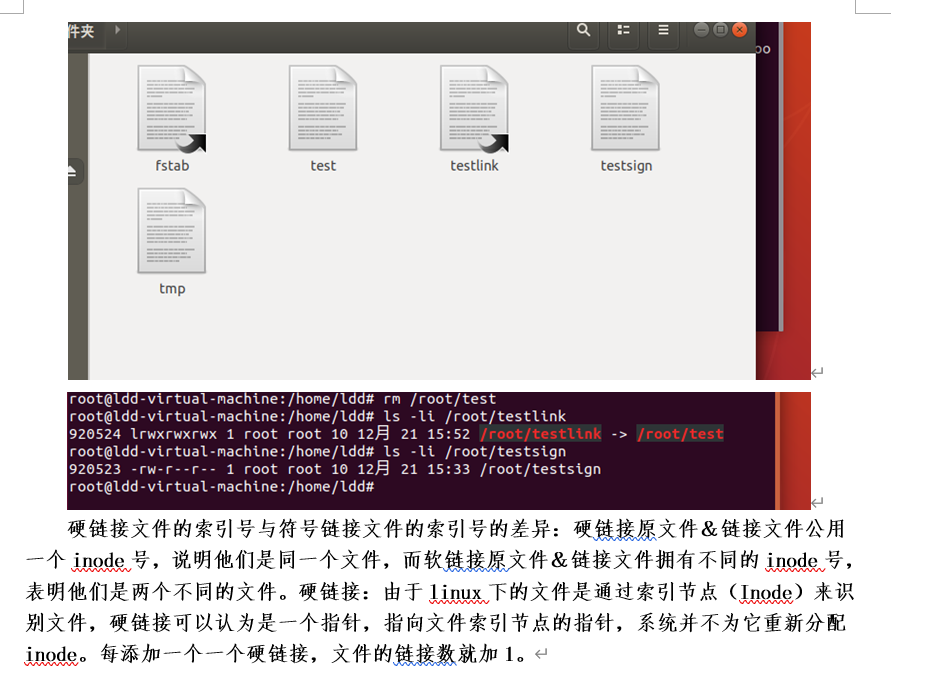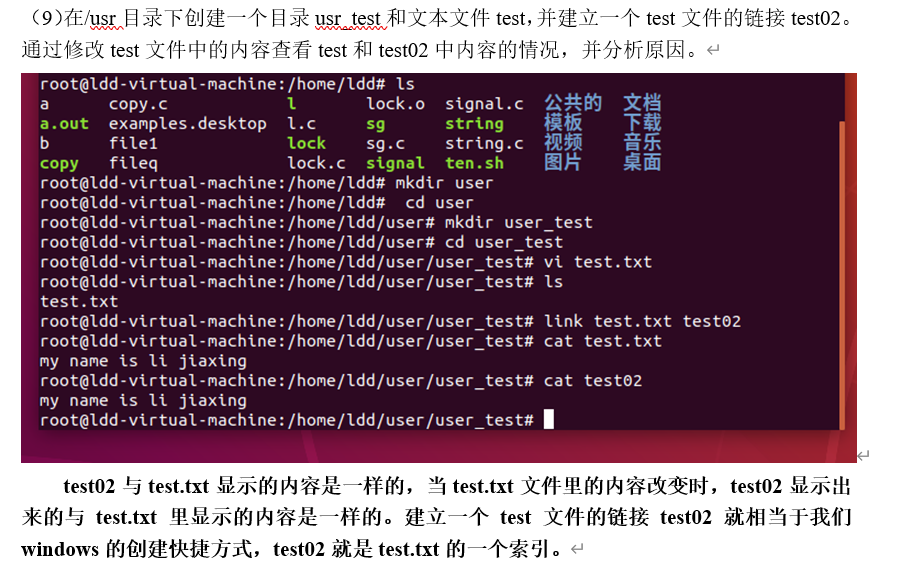四、编程实验结果截图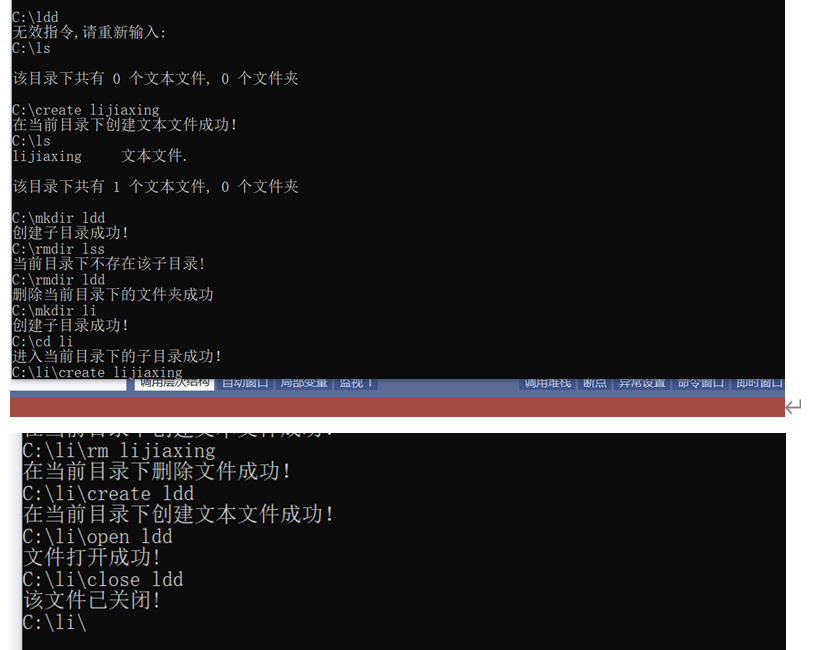五、实验心得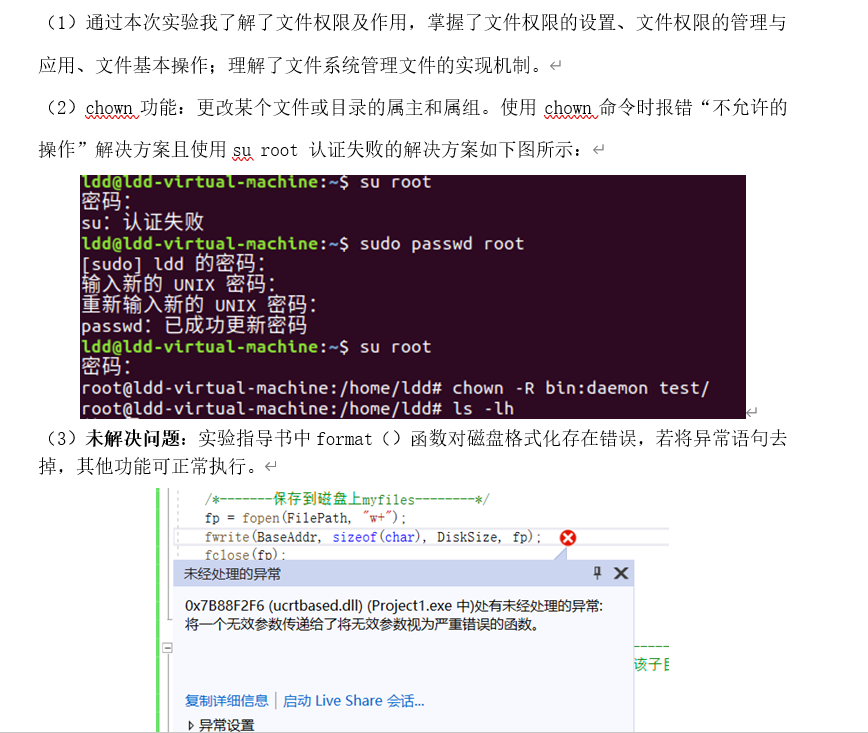六、附件（编程实验实验代码）：（来源百度文库，当时是下载的百度文库资源，如侵权请留言

#include <stdio.h>
#include <memory.h>
#include <string>
#include <iostream>
using namespace std;
#define GENERAL 1//1代表普通文件2代表目录文件0表示空文件
#define DIRECTORY 2
#define Zero 0
struct FCB
{
char fname; //文件名
char type;  // 0空文件  1目录文件 2空文件
int size;    //文件大小
int fatherBlockNum;    //当前的父目录盘块号
int currentBlockNum;    //当前的盘块
void initialize()
{
strcpy(fname, "\0");
type = Zero;
size = 0;
fatherBlockNum = currentBlockNum = 0;
}
};
const char* FilePath = "D:\\";/*常量设置*/
const int BlockSize = 512;       //盘块大小
const int OPEN_MAX = 5;          //能打开最多的文件数
const int BlockCount = 128;   //盘块数
const int DiskSize = BlockSize * BlockCount;    //磁盘大小
const int BlockFcbCount = BlockSize / sizeof(FCB);//目录文件的最多FCB数
int OpenFileCount = 0; // 统计当前打开文件数目
struct OPENLIST      //用户文件打开表
{
int files;      //当前打开文件数
FCB f[OPEN_MAX];    //FCB拷贝
OPENLIST()
{
files = 0;
for (int i = 0; i < OPEN_MAX; i++) {
f[i].fatherBlockNum = -1;//为分配打开
f[i].type = GENERAL;
}
}
};
struct dirFile/*-------------目录文件结构---------------*/
{
struct FCB fcb[BlockFcbCount];
void init(int _FatherBlockNum, int _CurrentBlockNum, char* name)//父块号，当前块号，目录名
{
strcpy(fcb.fname, name); //本身的FCB
fcb.fatherBlockNum = _FatherBlockNum;
fcb.currentBlockNum = _CurrentBlockNum;
fcb.type = DIRECTORY;     //标记目录文件
for (int i = 1; i < BlockFcbCount; i++) {
fcb[i].fatherBlockNum = _CurrentBlockNum; //标记为子项
fcb[i].type = Zero;    // 标记为空白项
}
}
};
struct DISK/**********************************************************************/
{
int FAT1[BlockCount];     //FAT1
int FAT2[BlockCount];     //FAT2
struct dirFile root;    //根目录
char data[BlockCount - 3][BlockSize];
void format() {
memset(FAT1, 0, BlockCount);     //FAT1
memset(FAT2, 0, BlockCount);     //FAT2
FAT1 = FAT1 = FAT1 = -2; //0,1,2盘块号依次代表FAT1,FAT2,根目录区
FAT2 = FAT2 = FAT2 = -2;  //FAT作备份
root.init(2, 2,(char*) "C:\\");//根目录区
memset(data, 0, sizeof(data));//数据区
}
};
FILE* fp;      //磁盘文件地址
string currentPath = "C:\\";   //当前路径
int current = 2;    //当前目录的盘块号
string cmd;      //输入指令
struct DISK* osPoint;    //磁盘操作系统指针
char command;    //文件名标识
struct OPENLIST* openlist; //用户文件列表指针
int  format();
int  mkdir(char* sonfname);
int rmdir(char* sonfname);
int create(char* name);
int listshow();
int delfile(char* name);
int changePath(char* sonfname);
int write(char* name);
int exit();
int open(char* file);
int close(char* file);
/*------------初始化-----------------------*/
int format()
{
current = 2;
currentPath = "C:\\";   //当前路径
osPoint->format();//打开文件列表初始化
delete openlist;
openlist = new OPENLIST;
/*-------保存到磁盘上--------*/
fp = fopen(FilePath, "w+");
fclose(fp);
printf("格式化成功！！\n");
return 1;
}
int  mkdir(char* sonfname)/*-----------------------创建子目录-------------------*/
{//判断是否有重名寻找空白子目录项寻找空白盘块号当前目录下增加该子目录项分配子目录盘块，并且初始化修改fat表
int i, temp, iFAT;
struct dirFile* dir;     //当前目录的指针
if (current == 2) // 根目录
dir = &(osPoint->root);
else
dir = (struct dirFile*)(osPoint->data[current - 3]);
/*--------为了避免该目录下同名文件夹--------*/
for (i = 1; i < BlockFcbCount; i++) {
if (dir->fcb[i].type == DIRECTORY && strcmp(dir->fcb[i].fname, sonfname) == 0) {
printf("该文件夹下已经有同名的文件夹存在了!\n");
return 0;
}
}
for (i = 1; i < BlockFcbCount; i++) {//查找空白fcb序号
if (dir->fcb[i].type == Zero)
break;
}
if (i == BlockFcbCount) {
printf("该目录已满!请选择新的目录下创建!\n");
return 0;
}
temp = i;
for (i = 3; i < BlockCount; i++) {
if (osPoint->FAT1[i] == 0)
break;
}
if (i == BlockCount) {
printf("磁盘已满!\n");
return 0;
}
iFAT = i;
/*-------------接下来进行分配----------*/
osPoint->FAT1[iFAT] = osPoint->FAT2[iFAT] = 2;   //2表示分配给下级目录文件
//填写该分派新的盘块的参数
strcpy(dir->fcb[temp].fname, sonfname);
dir->fcb[temp].type = DIRECTORY;
dir->fcb[temp].fatherBlockNum = current;
dir->fcb[temp].currentBlockNum = iFAT;
//初始化子目录文件盘块
dir = (struct dirFile*)(osPoint->data[iFAT - 3]);   //定位到子目录盘块号
dir->init(current, iFAT, sonfname);//iFAT是要分配的块号，这里的current用来指要分配的块的父块号
printf("创建子目录成功！\n");
return 1;
}
int rmdir(char* sonfname)/*-------删除当前目录下的文件夹--------*/
{
int i, temp, j;//确保当前目录下有该文件,并记录下该FCB下标
struct dirFile* dir;     //当前目录的指针
if (current == 2)
dir = &(osPoint->root);
else
dir = (struct dirFile*)(osPoint->data[current - 3]);
for (i = 1; i < BlockFcbCount; i++) {     //查找该目录文件
if (dir->fcb[i].type == DIRECTORY && strcmp(dir->fcb[i].fname, sonfname) == 0) {
break;
}
}
temp = i;

if (i == BlockFcbCount) {
printf("当前目录下不存在该子目录!\n");
return 0;
}
j = dir->fcb[temp].currentBlockNum;
struct dirFile* sonDir;     //当前子目录的指针
sonDir = (struct dirFile*)(osPoint->data[j - 3]);

for (i = 1; i < BlockFcbCount; i++) { //查找子目录是否为空目录
if (sonDir->fcb[i].type != Zero)
{
printf("该文件夹为非空文件夹,为确保安全，请清空后再删除!\n");
return 0;
}
}
/*开始删除子目录操作*/
osPoint->FAT1[j] = osPoint->FAT2[j] = 0;     //fat清空
char* p = osPoint->data[j - 3];      //格式化子目录
memset(p, 0, BlockSize);
dir->fcb[temp].initialize();          //回收子目录占据目录项
printf("删除当前目录下的文件夹成功\n");
return 1;
}
/*-----------在当前目录下创建文本文件---------------*/
int create(char* name) {
int i, iFAT;//temp,
int emptyNum = 0, isFound = 0;        //空闲目录项个数
struct dirFile* dir;     //当前目录的指针
if (current == 2)
dir = &(osPoint->root);
else
dir = (struct dirFile*)(osPoint->data[current - 3]);
for (i = 1; i < BlockFcbCount; i++)//查看目录是否已满//为了避免同名的文本文件
{
if (dir->fcb[i].type == Zero && isFound == 0) {
emptyNum = i;
isFound = 1;
}
else if (dir->fcb[i].type == GENERAL && strcmp(dir->fcb[i].fname, name) == 0) {
printf("无法在同一目录下创建同名文件!\n");
return 0;
}
}
if (emptyNum == 0) {
printf("已经达到目录项容纳上限，无法创建新目录!\n");
return 0;
}
for (i = 3; i < BlockCount; i++)//查找FAT表寻找空白区，用来分配磁盘块号j
{
if (osPoint->FAT1[i] == 0)
break;
}
if (i == BlockCount) {
printf("磁盘已满!\n");
return 0;
}
iFAT = i;
/*------进入分配阶段---------*///分配磁盘块
osPoint->FAT1[iFAT] = osPoint->FAT2[iFAT] = 1;
/*-----------接下来进行分配----------*///填写该分派新的盘块的参数
strcpy(dir->fcb[emptyNum].fname, name);
dir->fcb[emptyNum].type = GENERAL;
dir->fcb[emptyNum].fatherBlockNum = current;
dir->fcb[emptyNum].currentBlockNum = iFAT;
dir->fcb[emptyNum].size = 0;
char* p = osPoint->data[iFAT - 3];
memset(p, 4, BlockSize);
printf("在当前目录下创建文本文件成功！\n");
return 1;
}
/*-------查询子目录------------*/
int listshow()
{
int i, DirCount = 0, FileCount = 0;
//搜索当前目录
struct dirFile* dir;     //当前目录的指针
if (current == 2)
dir = &(osPoint->root);
else
dir = (struct dirFile*)(osPoint->data[current - 3]);
for (i = 1; i < BlockFcbCount; i++) {
if (dir->fcb[i].type == GENERAL) {   //查找普通文件
FileCount++;
printf("%s     文本文件.\n", dir->fcb[i].fname);
}
if (dir->fcb[i].type == DIRECTORY) {   //查找目录文件
DirCount++;
printf("%s     文件夹.\n", dir->fcb[i].fname);
}
}
printf("\n该目录下共有 %d 个文本文件, %d 个文件夹\n\n", FileCount, DirCount);
return 1;
}
/*---------在当前目录下删除文件-----------*/
int delfile(char* name)
{
int i, temp, j;
//确保当前目录下有该文件,并且记录下它的FCB下标
struct dirFile* dir;     //当前目录的指针
if (current == 2)
dir = &(osPoint->root);
else
dir = (struct dirFile*)(osPoint->data[current - 3]);
for (i = 1; i < BlockFcbCount; i++) //查找该文件
{
if (dir->fcb[i].type == GENERAL && strcmp(dir->fcb[i].fname, name) == 0) {
break;
}
}
if (i == BlockFcbCount) {
printf("当前目录下不存在该文件!\n");
return 0;
}
int k;
for (k = 0; k < OPEN_MAX; k++) {
if ((openlist->f[k].type = GENERAL) &&
(strcmp(openlist->f[k].fname, name) == 0)) {
if (openlist->f[k].fatherBlockNum == current) {
break;
}
else {
printf("该文件未在当前目录下!\n");
return 0;
}
}
}
if (k != OPEN_MAX) {
close(name);
}
//从打开列表中删除	/*开始删除文件操作*/
temp = i;
j = dir->fcb[temp].currentBlockNum;    //查找盘块号j
osPoint->FAT1[j] = osPoint->FAT2[j] = 0;     //fat1,fat2表标记为空白
char* p = osPoint->data[j - 3];
memset(p, 0, BlockSize); //清除原文本文件的内容
dir->fcb[temp].initialize();    //type=0;     //标记该目录项为空文件
printf("在当前目录下删除文件成功！\n");
return 1;
}
/*--------------进入当前目录下的子目录--------------*/
int changePath(char* sonfname)
{
struct dirFile* dir;     //当前目录的指针
if (current == 2)
dir = &(osPoint->root);
else
dir = (struct dirFile*)(osPoint->data[current - 3]);
/*回到父目录*/
if (strcmp(sonfname, "..") == 0) {
if (current == 2) {
printf("你现已经在根目录下!\n");
return 0;
}
current = dir->fcb.fatherBlockNum;
currentPath = currentPath.substr(0, currentPath.size() - strlen(dir->fcb.fname) - 1);
return 1;
}
/*进入子目录*///确保当前目录下有该目录,并且记录下它的FCB下标
int i, temp;
for (i = 1; i < BlockFcbCount; i++) {     //查找该文件
if (dir->fcb[i].type == DIRECTORY && strcmp(dir->fcb[i].fname, sonfname) == 0) {
temp = i;
break;
}
}
if (i == BlockFcbCount) {
printf("不存在该目录!\n");
return 0;
}
//修改当前文件信息
current = dir->fcb[temp].currentBlockNum;
currentPath = currentPath + dir->fcb[temp].fname + "\\";
printf("进入当前目录下的子目录成功！\n");
return 1;
}
int exit() {//保存到磁盘上C:\myfiles
//将所有文件都关闭/*--------System exit---------------------*/
fp = fopen(FilePath, "w+");
fclose(fp);
//释放内存上的虚拟磁盘
free(osPoint);
//释放用户打开文件表
delete openlist;
printf("退出文件系统成功！\n\n");
return 1;
}
int write(char* name)/*-------------在指定的文件里记录信息---------------*/
{
int i;
char* startPoint, * endPoint;
//在打开文件列表中查找 file(还需要考虑同名不同目录文件的情况!!!)
for (i = 0; i < OPEN_MAX; i++) {
if (strcmp(openlist->f[i].fname, name) == 0) {
if (openlist->f[i].fatherBlockNum == current) {
break;
}
else {
printf("该文件处于打开列表中，本系统只能改写当前目录下文件!\n");
return 0;
}
}
}
if (i == OPEN_MAX) {
printf("该文件尚未打开,请先打开后写入信息!!\n");
return 0;
}
int active = i;
int fileStartNum = openlist->f[active].currentBlockNum - 3;
startPoint = osPoint->data[fileStartNum];
endPoint = osPoint->data[fileStartNum + 1];
printf("请输入文本以Ctrl D号结束:\t");
char input;
while (((input = getchar()) != 4)) {
if (startPoint < endPoint - 1) {
*startPoint++ = input;
}
else {
printf("达到单体文件最大容量！");
*startPoint++ = 4;
break;
}
}
return 1;
}
{
int i, fileStartNum;
char* startPoint, * endPoint;
//struct dirFile *dir;
//在打开文件列表中查找 file(还需要考虑同名不同目录文件的情况!!!)
for (i = 0; i < OPEN_MAX; i++) {
if (strcmp(openlist->f[i].fname, file) == 0) {
if (openlist->f[i].fatherBlockNum == current) {
break;
}
else {
printf("该文件处于打开列表中，本系统只能阅读当前目录下文件!\n");
return 0;
}
}
}
if (i == OPEN_MAX) {
printf("该文件尚未打开,请先打开后读取信息!\n");
return 0;
}
int active = i;//计算文件物理地址
fileStartNum = openlist->f[active].currentBlockNum - 3;
startPoint = osPoint->data[fileStartNum];
endPoint = osPoint->data[fileStartNum + 1];
printf("该文件的内容为:  ");
while ((*startPoint) != 4 && (startPoint < endPoint)) {
putchar(*startPoint++);
}
printf("\n");
return 1;
}
int open(char* file)//打开文件/*当前目录下添加一个打开文件*/
{
int i, FcbIndex;
//确保没有打开过该文件 = 相同名字 + 相同目录
for (i = 0; i < OPEN_MAX; i++) {
if (openlist->f[i].type == GENERAL && strcmp(openlist->f[i].fname, file) == 0
&& openlist->f[i].fatherBlockNum == current)
{
printf("该文件已经被打开!\n");
return 0;
}
}
//确保有空的打开文件项
if (openlist->files == OPEN_MAX) {
printf("打开文件数目达到上限!无法再打开新文件.\n");
return 0;
}
//确保当前目录下有该文件,并且记录下它的FCB下标
struct dirFile* dir;     //当前目录的指针
if (current == 2)
dir = &(osPoint->root);
else
dir = (struct dirFile*)(osPoint->data[current - 3]);
for (i = 1; i < BlockFcbCount; i++) {     //查找该文件
if (dir->fcb[i].type == GENERAL && strcmp(dir->fcb[i].fname, file) == 0) {
FcbIndex = i;
break;
}
}
if (i == BlockFcbCount) {
printf("当前目录下不存在该文件!\n");
return 0;
}
//装载新文件进入打开文件列表,(FCB信息，文件数++)
openlist->f[OpenFileCount] = dir->fcb[FcbIndex]; //FCB拷贝
openlist->files++;
printf("文件打开成功!\n");
OpenFileCount++;
return 1;
}
int close(char* file)
{
//释放该文件所占内存//释放用户打开文件列表表项
int i;
//在打开文件列表中查找 file(还需要考虑同名不同目录文件的情况!!!)
for (i = 0; i < OPEN_MAX; i++) {
if ((openlist->f[i].type = GENERAL) &&
(strcmp(openlist->f[i].fname, file) == 0)) {
if (openlist->f[i].fatherBlockNum == current) {
break;
}
else {
printf("该文件已打开，但未在当前目录下，无法关闭!\n");
return 0;
}
}
}
if (i == OPEN_MAX) {
printf("该文件未在打开列表中!\n");
return 0;
}
int active = i;
openlist->files--;
openlist->f[active].initialize();
OpenFileCount--;
printf("该文件已关闭!\n");
return 1;
}
int main()
{
printf("@ Welcome To My Operate System Of File(FAT)         @n");
printf("\n  以下是使用说明书：\n");//使用说明书
printf("@@@@@@@@@@@@@@@@@@@@@@@@@@@@@@@@@@@@@@@@@@@\n");
printf("@ format :对磁盘格式化.                             @ \n");
printf("@ exit   :安全退出该文件系统,保存信息.              @ \n");
printf("@ mkdir dirname :创建子目录.                        @ \n");
printf("@ rmdir dirname :删除子目录.                        @ \n");
printf("@ ls    dirname :显示当前目录下信息.                @ \n");
printf("@ cd     dirname :更改当前目录.                     @ \n");
printf("@ create filename :创建一个新文件,并且打开.         @ \n");
printf("@ write filename :选择一个打开的文件写入信息        @ \n");
printf("@ read   filename :选择一个打开的文件读取信息.      @ \n");
printf("@ rm     filename :删除文件.                        @ \n");
printf("@ open   filename :打开文件.                        @\n");
printf("@ close filename :关闭文件.                         @\n");
printf("@@@@@@@@@@@@@@@@@@@@@@@@@@@@@@@@@@@@@@@@@@\n\n");
openlist = new OPENLIST;//创建用户文件打开表
if ((fp = fopen(FilePath, "r")) != NULL) {//加载磁盘文件
printf("加载磁盘文件( %s )成功,现在可以进行操作了!\n\n", FilePath);
}
else {
printf("这是你第一次使用该文件管理系统!\t正在初始化...\n");
format();
printf("初始化已经完成,现在可以进行操作了!\n\n");
}
while (1) {
cout << currentPath;
cin >> cmd;
if (cmd == "format") {
format();
}
else if (cmd == "mkdir") {
cin >> command;
mkdir(command);
}
else if (cmd == "rmdir") {
cin >> command;
rmdir(command);
}
else if (cmd == "ls") {
listshow();
}
else if (cmd == "cd") {
cin >> command;
changePath(command);
}
else if (cmd == "create") {
cin >> command;
create(command);
}
else if (cmd == "write") {
cin >> command;
write(command);
}
else if (cmd == "read") {
cin >> command;
}
else if (cmd == "rm") {
cin >> command;
delfile(command);
}
else if (cmd == "open") {
cin >> command;
open(command);
}
else if (cmd == "close") {
cin >> command;
close(command);
}
else if (cmd == "exit") {
exit();
break;
}
else cout << "无效指令,请重新输入:" << endl;
}
printf("Thank you for using my file system!\n");
return 1;
}

展开全文linux 操作系统
• 《操作系统实验5文件系统_Linux文件管理》由会员分享，可在线阅读，更多相关《操作系统实验5文件系统_Linux文件管理(14页珍藏版)》请在人人文库... (3 )了解操作系统文件系统的工作原理和工作方式。 2 实验内容 (1 ...

《操作系统实验5文件系统_Linux文件管理》由会员分享，可在线阅读，更多相关《操作系统实验5文件系统_Linux文件管理(14页珍藏版)》请在人人文库网上搜索。

1、实验 5 文件系统：Linux 文件管理 1 实验目的 (1 )掌握 Linux 提供的文件系统调用的使用方法； (2 )熟悉文件和目录操作的系统调用用户接口； (3 )了解操作系统文件系统的工作原理和工作方式。 2 实验内容 (1 ) 利用 Linux 有关系统调用函数编写一个文件工具filetools ，要求具有下列功能： * 0. 退出 1. 创建新文件 2. 写文件 3. 读文件 4. 复制文件 5. 修改文件权限 6. 查看文件权限 7. 创建子目录 8. 删除子目录 9. 改变当前目录到指定目录 10. 链接操作 * 代码 ： #include .专业 .专注. . #includ。

2、e #include #include #include #include #include #include void menu(void); void openfile(void); voidwritefile(void); void readfile(void); void copyfile(void); void chmd(void); void ckqx(void); void cjml(void); void scml(void); void ggml(void); void ylj(void); int main() int choose; int suliangjin=1; .。

3、专业 .专注. . menu(); scanf(%d, while(choose!=0) switch(choose) case 1:openfile();break; case 2:writefile();break; case 3:readfile();break; case 4:copyfile();break; case 5:chmd();break; case 6:ckqx();break; case 7:cjml();break; case 8:scml();break; case 9:ggml();break; case 10:ylj();break; menu(); scanf。

4、(%d, return 0; .专业 .专注. . void menu(void) printf(文件系统 n); printf(1.创建新文件 n); printf(2.写文件 n); printf(3.读文件 n); printf(4.复制文件 n); printf(5.修改文件权限 n); printf(6.查看文件权限 n); printf(7.创建子目录 n); printf(8.删除子目录 n); printf(9.改变目前目录到指定目录n); printf(10.链接操作 n); printf(0.退出 n); printf(请输入您的选择.n); void openfile(v。

5、oid) int fd; if(fd=open(/tmp/hello.c,O_CREAT|O_TRUNC|O_RDWR,0666)0) perror(open); .专业 .专注. . else printf(open file:hileo.c%dn,fd); if(close(fd)0) perror(close); else printf(Close ); voidwritefile(void) int fd,size,len; char *buf=Hello!Im writing to this file!; len=strlen(buf); if(fd=open(/tmp/hello.。

6、c,O_CREAT|O_TRUNC|O_RDWR,0666)0) perror(open); else printf(open file:hileo.c%dn,fd); if(size=write(fd,buf,len)0) perror(write); else printf(Write:%sn,buf); if(close(fd)0) perror(close); .专业 .专注. . else printf(Close ); void readfile(void) int fd,size; char b10; if(fd=open(/tmp/hello.c,O_CREAT|O_TRUNC。

7、|O_RDWR,0666)0) perror(open); else printf(open file:hileo.c%dn,fd); lseek(fd,0,SEEK_SET); if(size=read(fd,b,10)0) perror(read); else printf(read from file:%sn,b); if(close(fd)0) perror(close); else printf(Close ); .专业 .专注. . void copyfile(void) if(fork()=0) execlp(/bin/cp,cp,/tmp/hello.c,/tmp/he.c,N。

8、ULL); else wait(0); printf(将 hello.c复制 he.c); void chmd(void) int a; printf(1.文件主可读可写可执行n); printf(2.文件主可读 n); printf(3.文件主可写 n); printf(4.文件主可执行 n); printf(请输入您的选项n); scanf(%d, switch(a) case 1:chmod(/tmp/hello.c,S_IRWXU);printf(ok!n);break; case 2:chmod(/tmp/hello.c,S_IRUSR);printf(ok!n);break; .专。

9、业 .专注. . case 3:chmod(/tmp/hello.c,S_IWUSR);printf(ok!n);break; case 4:chmod(/tmp/hello.c,S_IXUSR);printf(ok!n);break; default:printf(您选择有误 n); void ckqx(void) char *path=/bin/ls; char *argv4=ls,-l,NULL; if(fork()=0) execv(path,argv); else wait(0); void cjml(void) if(mkdir(/tmp/a,S_IRWXU)0) perror(M。

10、kdir); else .专业 .专注. . printf(创建成功 n); void scml(void) if(rmdir(/tmp/a)0) perror(Rmdir); else printf(删除成功 n); void ggml(void) if(chdir(/tmp/bc)0) perror(chdir); else printf(更改目录成功 n); void ylj(void) if(link(hello.c,h.c)0) perror(Link); else printf(建立硬连接 n); .专业 .专注. . 打开文件 ： 写文件 ： 读文件 ： 复制文件 ： .专业 .。

11、专注. . 修改权限 : 查看权限 ： 创建目录 ： .专业 .专注. . 删除目录 ： 更改目录 ： 硬连接 ： 问题解决方案 ： 1. 在复制文件时用 execlp 函数 ，虽然有复制成功但是他就跳出整个程序 ，这就是一个缺 陷。后来我在 execlp 前面加 if(fork) () =0) 就可以咯 2. 硬连接不成功 ，老是抛出错误信息 ，后来发现原来是因为我的代码出错 ，还有我之前 .专业 .专注. . 已经更改了目录所以就会经常报错 3.到现在还不知道为什么我读出来的是乱码情解决 实验心得体会 ： 通过这次实验 掌握Linux提供的文件系统调用的使用方法；熟悉文件和目录操作的调 用用户接口 ，了解操作系统文件系统的工作原理和工作方式。 .专业 .专注.。

展开全文• 操作系统实验(文件目录管理java实现)操作系统实验(文件目录管理java实现)一 实验目的1 深刻理解文件目录管理的概念。2 掌握文件目录管理问题，文件系统的树形结构。二 实验设备PC机三 实验内容在Java开发平台模拟...java文件管理实验
• 操作系统实验七Linux下的文件管理操作系统实验七：Linux下的文件管理一.实验目的1.熟悉Linux 下常用的操作指令。2.加深对文件，目录，文件系统等概念的理解。3.掌握Linux 文件系统的目录结构。4.掌握有关Linux 文件...
• 操作系统实验文件系统的用户界面实验目的进一步理解、使用和掌握文件的系统调用、文件的标准子例程，能利用和选择这些基本的文件操作完成复杂的文件处理工作。实验题目1．编写一个文件复制的C语言程序：分别使用...
• (3)了解操作系统文件系统的工作原理和工作方式。2．实验内容(1)利用Linux有关系统调用函数编写一个文件工具filetools，要求具有下列功能：***********0. 退出1. 创建新文件2. 写文件3. 读文件4. 复制文件5. 修改文件...
• 1、实验5-操作系统进程与文件管理-实验报告表-x(本文档包含：实验5实验报告 与 第5周作业题)实验5操作系统进程与文件管理实验报告学号 1404505147 姓名 榭雨骅 班级： 临床医学14 ( 1 )实验时间： xxx...
• 操作系统实验一、二实验报告实验目的熟悉Linux系统，掌握Linux系统的登入、退出等操作；熟悉Linux命令及进程管理；学会使用Linux下C语言编程的基本知识；加深对进程概念的理解，明确进程和程序的区别；进一步认识...
• 1 实验目的了解文件管理系统的作用和工作方式了解FAT文件系统的结构学习文件管理系统的一般开发方法。2 需求说明2.1 基本要求设计并实现一个目录列表函数(无须支持选项，如ls -a、ls -l等)，用来显示当前目录下包含...
• 《Linux操作系统》课程实验报告实验4 文件和目录操作一、实验目的1、理解Linux文件系统的结构和目录组织方式；2、掌握Linux常用目录和文件命令的使用。二、实验内容与要求以root用户身份登录Linux1、Linux目录管理...
• 一、实验目的1、熟悉Linux文件系统文件和目录结构，掌握Linux文件系统的基本特征；2、模拟实现Linux文件系统的简单I/O流操作：备份文件。二、实验环境Linux系统三、实验内容1、浏览Linux系统根目录下的子目录，...
• 文档介绍：实验4存储管理班级:姓名:学号:Windows内存结构本节实验的目的是:1)通过实验了解Windows内存的使用,学****如何在应用程序中管理内存,体会Windows应用程序内存的简单性和自我防护能力。2)了解Windows的内存...
• 操作系统实验一 Linux基本环境及进程管理实验一 Linux基本环境一、实验目的(1)熟悉Linux下的基本操作，学会使用各种Shell命令去操作Linux，对Linux有一个感性认识。(2)学会使用vi编辑器编辑简单的C语言程序，并能对...
• 《进程管理操作系统linux实验(20210313190726)》由会员分享，可在线阅读，更多相关《进程管理操作系统linux实验(20210313190726)(10页珍藏版)》请在人人文库网上搜索。1、实验 进程 管理一 ) 进程 的 创建实验实验...
• 文件系统-代码阅读并调试实验 二、实验目的 1、阅读下面源代码，写出程序功能。 2、阅读代码，分析代码中算法并说明。 3、调试并运行代码，写出结果。 源程序如下： #define _CRT_SECURE_NO_WARNINGS 1 #include&...操作系统 c++
• Linux实验4-Linux文件系统-目录和文件管理实 验 报 告课程名称： Linux操作系统实验名称：实验4、Linux文件系统-目录和文件管理学 生 姓 名： 班 级 学 号学 院(系)： 指 导 教 师：实验时间： 年 月 日 实验成绩：...
• GDOU-B-11-112广东海洋大学学生实验报告书(学生用表)GDOU-B-11-112实验名称Linux进程基本管理课程名称计算机操作系统课程号学院(系)专业统班级学生姓名学号实验地点实验日期实验三 Linux进程基本管理一、实验目的...
• 编写程序，将指定文件的某个属性进行修改，属性可以是创建时间、最近一次访问时间以及最近一次修改的时间等基本属性中任意一个。 #include<stdio.h> #include<windows.h> #include<iostream> #...
• 操作系统实验五 设备管理实验五 Linux磁盘设备管理一.???? 实验目的：1.???????? 掌握磁盘分区的方法；2.???????? 掌握文件系统的建立、挂装、解挂和检查方法；3.???????? 理解自动挂装；二.???? 实验内容：1.??????...
• 目录前言实验内容实验实验实验实验四 前言 由于是小组实验，我分配被问到的是实验三的sender线程的实现，因此只记录了这一块内容可能问到的问题，不过代码里的注释很详尽了，可以自行观看。 实验内容 （1）...操作系统 linux 实验 进程管理
• 《《实用操作系统实验报告五linux设备管理》由会员分享，可在线阅读，更多相关《《实用操作系统实验报告五linux设备管理(16页珍藏版)》请在人人文库网上搜索。1、实用操作系统实验报告实验报告： 5 实验项目名称...
• linux 实验报告 文件系统及文件管理学生课程实验报告书课程： 《linux网络操作系统》级 系专业 班学号：姓名：指导教师：一、实验项目： 用户与组群管理二、实验日期：三、实验原理：1.熟悉Linux下常用的操作指令。2...
• 目录前言参考文献代码 ...因为代码量较大，建议先把文件系统的逻辑以及各个专业术语的概念如FCB、FAT、用户打开文件表等搞懂再看代码，概念可以看书上p352的预备知识，或者看这篇博客：文件结构。 看完之后再手操作系统 linux 文件系统 实验
• 内存管理一、实验目的二、...操作系统的一项主要任务对一个进程的内存区域进行保护，不允许其他进程访问。例如，你的网页浏览器不应该干扰你的文本编辑器。为了实现这一目标，有不同的方法，x86上，硬件支持两种不同的操作系统
• 精品文档.(本文档包含： 实验5 实验报告 与 第5周作业题)实验5 操作系统进程与文件管理 实验报告学号 1404505147 姓名禤雨骅 班级： 临床医学14(1) 实验时间：2015 年 04 月 13 日实验报告表 5 – SEQ 实验报告表_5_...
• 《linux操作系统实验七-Linux存储管理操作实践》由会员分享，可在线阅读，更多相关《linux操作系统实验七-Linux存储管理操作实践(7页珍藏版)》请在人人文库网上搜索。1、操作系统实验报告实验序号： 7 实验项目名称......